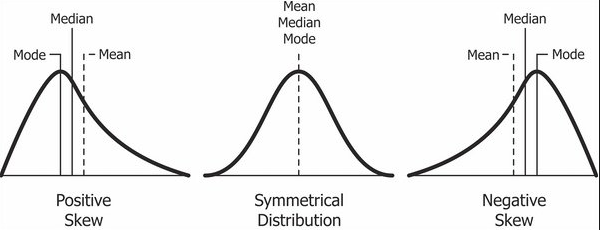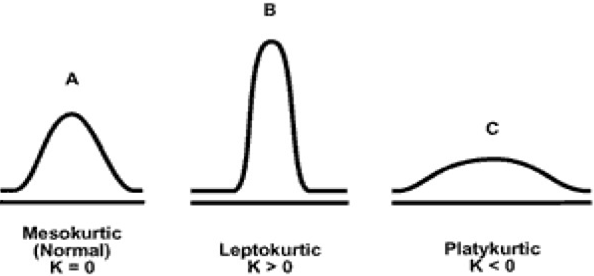• 0

# Skewness and Kurtosis

Go to solution Solved by vishwadeepak.choudhary26,

Skewness and Kurtosis

Skewness is the measure of asymmetry in a statistical distribution or a comparative measure of the two tails. If the curve is symmetrical, skewness will be zero. Right skewed distributions (longer right tail) will have a positive skew while left skewed distributions (longer left tail) will have a negative skew. Its values typically ranges from -3 to 3.

Kurtosis is a measure of sharpness of the peak of a distribution. It also gives us a measure of the combined weight of the tails as compared to the weight of the remaining part of the distribution. If the weight of tails is large, it means the curve will look flatter while if the weight is less, the curve will look like a sharp peak. A standard normal distribution has a Kurtosis of 3.

An application oriented question on the topic along with responses can be seen below. The best answer was provided by Vishwadeepak Choudhary on 27th August 2018.

## Question

Q. 87  Skewness and kurtosis are two commonly listed values when you run any software’s descriptive statistics function. The skewness parameter measures the relative sizes of the two tails. The kurtosis parameter is a measure of the combined weight of the tails relative to the rest of the distribution. Since these numbers appear automatically, it is natural to wonder how they might be used in practice. What purpose do these shape statistics serve in any data analysis?

Note for website visitors - Two questions are asked every week on this platform. One on Tuesday and the other on Friday.

## Recommended Posts

• 0
• Solution

Skewness and Kurtosis – the shape parameters (we can also call them tail parameters as both describe the tails of the distribution), as they are called so do they do. Skewness is the measure of the symmetry of the distribution or more precisely the lack of symmetry of the distribution. If your distribution is completely symmetrical, then you should get Skewness statistic as zero when you run the descriptive analysis. Skewness is also stated as a comparative measurement of the two tails of the distribution, if they are same the Skewness value would be zero.  Below picture exemplifies Symmetric (in the center) and asymmetric (left and right) distributions.If your data is skewed towards the right, then it is called positive Skewness and if it is skewed towards left, then it is called negative Skewness. So now, when your statistical software throws a (–ve) or (+ve) number, you know what it is. We all know that normal (bell curve) distribution is symmetric so the Skewness parameter for a normal distribution is zero.

Let’s talk about Kurtosis. In the beginning, it was understood as a measure of peakedness of the distribution but this was later proven not to be correct. There does exist some correlation between Kurtosis and peakedness but more research is required to comment further on the relationship. Kurtosis gives us the combined weight of the tails as compared to the weight of the remaining part of the distribution. A normal distribution would have a Kurtosis parameter of 3. Many software packages throw another output for Kurtosis which is called Excess Kurtosis. Excess Kurtosis is the relative Kurtosis from a normal distribution thus excess Kurtosis for a normal distribution is zero. We have three more little terrifying terms here: Platykurtic, Mesokurtic and Leptokurtic. Platykurtic distribution is with excess Kurtosis of less than zero (-ve), Mesokurtic distribution is with excess Kurtosis of zero (0) and Leptokurtic distributions is with excess Kurtosis of more than zero (+ve). Refer to below image:We would talk about the usage of these two parameters now. As explained above, these definitely help us to know about the shapes of the distribution; more importantly whether we are working with normal distribution or not. However, these parameters are highly sensitive to the sample size that we are working on and changes drastically when the sample size is increased or decreased (as they are third and fourth standardized central moment of the model in analysis whereas standard deviation is only second standardized central moment). Thus, it’s difficult to rely on these statistics (or parameters). You can always deduce information about the shape of the distribution through your mean (location) and standard deviation (dispersion) summaries. I would like to re-iterate what Dr. Donald J. Wheeler mentions on these parameters:

"In short, skewness and kurtosis are practically worthless. Shewhart made this observation in his first book. The statistics for skewness and kurtosis simply do not provide any useful information beyond that already given by the measures of location and dispersion."

As a conclusion, there appears very little reason why we should bother about the Skewness and Kurtosis parameters. The histogram, location, and dispersion should be able to help us with the information that we need.

##### Share on other sites
• 0

One of the most important thing that one would like to infer from a descriptive statistics output for any data is how much does the data distribution comply or deviate from a normal distribution.

Skewness and Kurtosis are measures that quantify such deviation, often referred to as measures for 'shape' related parameters. These measures will be particularly useful while comparing 2 distributions, and decide on the extent of normality - For eg. the delivery time for a product when compared between two delivery outlets.

Data may be distributed either spread out more on left or on the right or uniformly spread. For a normal distribution, the data will be spread uniformly about a central point, and not skewed. When the data is scattered uniformly at the central point, it called as Normal Distribution. Here median, mode and mean are at the same point and the skewness is zero. When skewness is negative, it means that the data is left skewed. If it is positive, then the data is said to be right skewed, as illustrated below. While the graphical representation provides a very quick and easily understandable comparison of the skewness or bias on the data distribution, the skewness measure helps in quantifying the same. This will be particularly important for decision making while comparing distributions which appear similar, but have smaller differences in skew that may not show up well on the graph.

In economics, the skewness measure is often used to study income distributions that are skewed to the right or to the left.

Data distributions based on life times of certain products, like a bulb or other electrical devices, are right skewed. The smallest lifetime may be zero, whereas the long lasting products will provide the positive skewness.

Kurtosis is often referred to as a measure of the 'pointedness' of the peak of the distribution. It is also referred as measure of  the 'weight of the tails' of distibution. However, I will attempt to make the understanding of Kurtosis better in as simple terms as possible. It is known that while Normal distributions are symmetrical in nature, not all symmetrical distributions are Normal. A perfect normal distribution will have a Kurtosis represented as β2–3 = 0. A positive kurtosis, known as Leptokurtic will have  β2–3 > 0; a negative kurtosis, known as Platykurtic will have β2–3 < 0. To illustrate with an example, most of us are familiar with 't' distribution, which will may appear seemingly similar to Normal distribution, but it will be differentiated by a β2–3 that will be greater than zero.

Though Kurtosis is mostly referred to with respect to the "peaked-ness" and "tail heaviness", it is really dependent on the extent of mass that moves to or from the 'center' of the distribution as compared with a normal distribution with same mean and variance.

One of the main uses of Kurtosis is to use it as underlying factor for testing Normality, since many of the statistical techniques depend on the normality of distribution.

##### Share on other sites
• 0

Given the skewness and Kurtosis we could predict the shape of a probability distribution.

Skewness:

The Lack of Symmetry in the probability distribution is called Skewness, A distribution is positive skewed when it has a long tail to the right (Right tail are + skewed) and a distribution is negative skewed if it has a long tail towards left. Further it is also interesting to know that when we check the data points using the Box plot if the mean of the dataset is greater that the median then its negative skewed and when the mean is less than median then its positive skewed.

Kurtosis:

The sharpness in the probability distribution is referred to as Kurtosis. Flatter curves are PlatyKurtic (-ve Kurtosis) and Sharper curves are  (+ve Kurtosis)

##### Share on other sites
• 0

The chosen best answer is that of Vishwadeepak Choudhary. The answer mentions correct definitions (please note that experts discarded the Kurtosis definition which mentions "peakedness" of data and only define it as the combined weight of the tails with respect to the rest of the distribution" ; the images  in the answer make it easy to understand the definitions. The answer also mentions correctly that the  measures are sensitive to sample size and do not provide any additional information than we would get from other tools and measures such as boxplot, center, dispersion etc.

Kudos to all three participants, this was a tricky question to attempt!

##### Share on other sitesThis topic is now closed to further replies.

• ### Forum Statistics

• Total Topics
2,867
• Total Posts
14,508
• ### Member Statistics

• Total Members
55,051
• Most Online
888